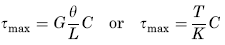Related Resources: calculators

### I Beam Section Torque Deflection, Stress Equation and Calculator

Beam Deflection and Stress Equation and Calculators

Torque Applied Deformation and Stress of a I Beam Section Equations and CalculatorALL calculators require a Premium Membership

Preview

Torque Applied Deformation and Stress of a I Beam Section Calculator

K = 2K1 + K2 + 2αD4

Where:t = b if b < d,
t = d if d < b,
t1 = b if b > d,
t1 = d if d > bAngle of Twist under applied Torque Moment

θ = ( T L ) / ( K G )

Shear Stress MaximumA = 2 a b + d c + 4 (πr2/4)

Where:

θ = angle of twist (radians),
α = degrees,
T = Twisting or torque moment force-length, (in-lbs, N-mm),
L = Length of Section (in, mm),
a, b, c, d = Length as Indicated (in, mm),
D = Diameter (in, mm),
A = area of section (in2, mm2)
τ = Unit shear stress force / area (lbs/in2, N/mm2),
G = Modulus of rigidity force / area (lbs/in2, N/mm2),
K = Polar Moment of Inertia (in4, mm4) for section

Reference:
Roarks Formulas for Stress and Strain, 7th Edition, Table 10.1 Formulas for torsional deformation and stress.